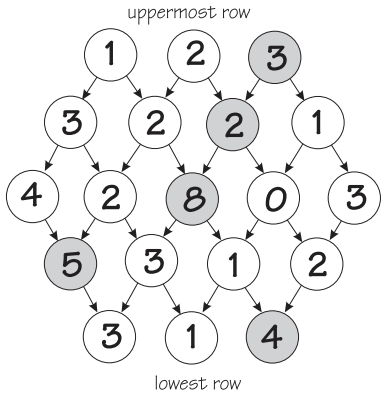시간 제한메모리 제한제출정답맞힌 사람정답 비율
1 초 128 MB111100.000%

## 문제

Figure 1 shows a honeycomb of numbers (side length of the honeycomb is 3). A route starts from some node in the uppermost row and ends at some node in the lowest row. From a node, the route can continue only diagonally down to the left or diagonally down to the right. When creating a route through the honeycomb, you are allowed to make at most one swap of two numbers on at most one horizontal row of the honeycomb. (Swapping essentially means that in one chosen row you are allowed to place the greatest number of that row to any position on the same row.) Your task is to write a program that calculates the highest sum of numbers on any route using the ability of swapping two numbers on a chosen row.Figure 1. A honeycomb of side length 3.

## 입력

The side length of the honeycomb is in the first row of the input. If the side length is n, the honeycomb consists of 2n − 1 rows. Numbers on the rows of the honeycomb are on the following 2n − 1 rows as follows.

## 출력

The highest sum is written as an integer in the output.

## 제한

• The numbers in the nodes are integers between 0 and 99.
• The side length of the honeycomb is an integer between 1 and 99.

## 예제 입력 1

3
1 2 3
3 2 2 1
4 2 8 0 3
5 3 1 2
3 1 4


## 예제 출력 1

22


## 힌트

In the Figure 1 the correct solution (3 + 2 + 8 + 5 + 4 = 22) is gray shaded. Notice that number ’5’ on the 4 th row (from the top) is swapped to the 3 rd position (from the left) on that row.